$\def\lefteqn#1{\rlap{\displaystyle{#1}}} \newcommand{\Hajek}{H\'{a}jek} \newcommand{\Hastad}{H{\aa}stad} \newcommand{\Pudlak}{Pudl\'{a}k} \newcommand{\Krajicek}{Kraj\'\i\v{c}ek} \newcommand{\Jerabek}{Je\v{r}\'{a}bek} \newcommand{\Kolodziejczyk}{Ko{\l}odziejczyk} \newcommand{\compfont}{\mathsf} \newcommand{\SIG}{\hat\Sigma^{\compfont b}_{#1}} \newcommand{\SIB}{\Sigma^{\compfont b}_{#1}} \newcommand{\SIGINFTY}{\Sigma^{\compfont #1}_{\infty}} \newcommand{\ASIGINFTY}{\mathcal{A}\Sigma^{\compfont #1}_{\infty}} \newcommand{\pSIB}{p\Sigma^{\compfont b}_{#1}} \newcommand{\PI}{\hat\Pi^{\compfont b}_{#1}} \newcommand{\PIB}{\Pi^{\compfont b}_{#1}} \newcommand{\DELT}{\hat\Delta^b_{#1}} \newcommand{\DELTB}{\bigtriangledown_{#1}} \newcommand{\BOOL}{\compfont B} \newcommand{\UNIV}{\compfont U} \newcommand{\EXIST}{\compfont E} \newcommand{\LEX}{\compfont L} \newcommand{\PLS}{\compfont{PLS}} \newcommand{\FP}{\compfont{FP}} \newcommand{\PTIME}{\compfont{P}} \newcommand{\NP}{\compfont{NP}} \newcommand{\NC}{\compfont {NC}} \newcommand{\coNP}{\compfont{co}-\compfont{NP}} \newcommand{\PH}{\compfont{PH}} \newcommand{\polylog}{\compfont{polylog}} \newcommand{\SigmaP}{\Sigma^{\compfont p}_{#1}} \newcommand{\SiP}{\Sigma^{\compfont p}_{#1}} \newcommand{\PiP}{\Pi^{\compfont p}_{#1}} \newcommand{\theoryfont}{\mathit} \newcommand{\BASIC}{\theoryfont{BASIC}} \newcommand{\LKB}{\theoryfont{LKB}} \newcommand{\LK}{\theoryfont{LK}} \newcommand{\EBASIC}{\theoryfont{EBASIC}} \newcommand{\IOpen}{\theoryfont{IOpen}} \newcommand{\LIOpen}{\theoryfont{LIOpen}} \newcommand{\TOCOMP}{\theoryfont{TOComp}} \newcommand{\TCOMP}{\theoryfont{TComp}^{#1}} \newcommand{\IDelta}{\mbox{\theoryfont{I}\Delta_0}} \newcommand{\TT}{\theoryfont{T}^{#1}_2} \newcommand{\TR}{\theoryfont{\hat{T}}^{#1}_2} \newcommand{\ST}{\theoryfont{S}^{#1}_2} \newcommand{\RT}{\theoryfont{R}^{#1}_2} \newcommand{\RR}{\theoryfont{\hat R}^{#1}_2} \newcommand{\CT}{\theoryfont{\hat{C}}^{#1}_2} \newcommand{\quasip}{\theoryfont{\{2^{ \mathbf{ \dot{(||\id||)} } } \} }} \newcommand{\RFN}{\theoryfont{RFN}} \newcommand{\BPR}{\theoryfont{BPR}} \newcommand{\BDC}{\theoryfont{BDC}} \newcommand{\LLIND}{\theoryfont{LLIND}} \newcommand{\LIND}{\theoryfont{LIND}} \newcommand{\IND}{\theoryfont{IND}} \newcommand{\COMP}{\theoryfont{COMP}} \newcommand{\REPL}{\theoryfont{REPL}} \newcommand{\BB}{\theoryfont{BB}} \newcommand{\open}{\theoryfont{open}} \newcommand{\WIT}{\theoryfont{WIT}} \newcommand{\mathfnfont}{\mathrm} \newcommand{\K}{\mathfnfont{K}} \newcommand{\cons}{\mathfnfont{cons}(#1)} \newcommand{\car}{\mathfnfont{car}(#1)} \newcommand{\cdr}{\mathfnfont{cdr}} \newcommand{\cond}{\mathfnfont{cond}} \newcommand{\PAD}{\mathfnfont{PAD}} \newcommand{\CAT}{\mathfnfont{CAT}} \newcommand{\LSP}{\mathfnfont{LSP}} \newcommand{\MSP}{\mathfnfont{\lfloor\frac{#1}{2^{#2}}\rfloor}} \newcommand{\MULT}{\mathfnfont{MULT}} \newcommand{\Interval}{\mathfnfont{#1 \in [#2, #3)}} \newcommand{\BLK}{\mathfnfont{BLK}} \newcommand{\BIT}{\mathfnfont{BIT}} \newcommand{\OUT}{\mathfnfont{OUT}} \newcommand{\DMSB}{\mathfnfont{DMSB}} \newcommand{\PREV}{\mathfnfont{PREV}} \newcommand{\bit}{\mathfnfont{BIT}(#1)} \newcommand{\bool}{\mathfnfont{bool}(#1)} \newcommand{\longBool}{\mathfnfont{lbool}(#1)} \newcommand{\Pair}{\mathfnfont{pair}(#1)} \newcommand{\NOP}{\mathfnfont{NOP}} \newcommand{\Seq}{\mathfnfont{Seq}} \newcommand{\RIGHT}{\mathfnfont{right}} \newcommand{\ispair}{\mathfnfont{ispair}} \newcommand{\Len}{\mathfnfont{Len}} \newcommand{\modtwo}{\mathfnfont{mod2}} \newcommand{\id}{\mathfnfont{id}} \newcommand{\cl}{\mathfnfont{cl}} \newcommand{\proj}{(#1)_{#2}} \newcommand{\vec}{\mathbf{#1}} \newcommand{\bd}{\mathfnfont{bd}} \newcommand{\LAST}{\mathfnfont{LAST}} \newcommand{\SUB}{\mathfnfont{SUB}} \newcommand{\ov}{\overline} \newcommand{\proves}{\vdash} \newcommand{\sequent}{\rightarrow} \newcommand{\monus}{\frac{\cdot}{ }} \newcommand{\bigvw}{\mathop{\mathchoice% {\makebox[0pt][l]{\displaystyle\bigvee}\mbox{\displaystyle\bigwedge}}% {\makebox[0pt][l]{\textstyle\bigvee}\mbox{\textstyle\bigwedge}}% {\makebox[0pt][l]{\scriptstyle\bigvee}\mbox{\scriptstyle\bigwedge}}% {\makebox[0pt][l]{\scriptscriptstyle\bigvee} \mbox{\scriptscriptstyle\bigwedge}}}\limits} \newcommand{\IFF}{\Leftrightarrow} \newcommand{\AND}{\mathrel{\land}} \newcommand{\OR}{\mathrel{\lor}} \newcommand{\NOT}{\neg} \newcommand{\IMP}{\supset} \newcommand{\DAND}{\wedge\!\!\!\!\wedge} \newcommand{\DOR}{\vee\!\!\!\!\vee} \newcommand{\GN}{\,\!^{\lceil}\!#1\,\!^{\rceil}} \newcommand{\HALF}{\lfloor\frac{1}{2}#1\rfloor} \newcommand{\DIV}{\lfloor\frac{#1}{#2}\rfloor} \newcommand{\LEQ}{\leq_l} \newcommand{\nat}{\mathbb{N}} \newcommand{\EQN}{\begin{eqnarray*}#1\end{eqnarray*}} \newcommand{\EQ}{\begin{eqnarray}#1\end{eqnarray}} \newcommand{\TQ}{\hbox{#1}} \newenvironment{proof}% {\medskip \noindent {\it Proof.} }{\Box} \newcommand{\ignore}{}$# Facial Expression Video Synthesis from the StyleGAN Latent Space

Lei Zhang
Chris Pollett (Presenting)
May, 2021

# Introduction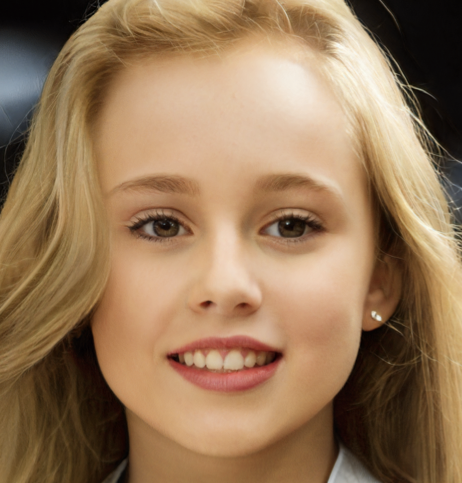• Given a still image such as to the right, we are interested in training a computer to make a video of what happens next.
• Here what happens next should be plausible to a human.
• To constrain the problem, we focus on facial starting images and restrict ourselves to emotion and pose changes for what happens next.
• For the rest of this talk, I'd like to briefly describe prior related work on computer video synthesis and then describe our system and some experiments we conducted with it.

# Prior Image Generation Systems 1

As with many video generation systems, our system make use of prior work on image generation:
• GANs (Generational Adversarial Networks) (Goodfellow, et al 2014) - have an image generator network which is trained alongside a discriminator network that tries to distinguish generator outputs from actual images.
• VGG (Visual Geometry Group) (Simonyan and Zisserman 2015) - train very deep CNNs by using small 3x3 kernels that perform well on ImageNet Challenge.
• Style Transfer (Gatys, et al 2016, Huang Belongi 2017) - work on transfer of style from one image to another, led to the idea of replacing batch normalization for style transfer to adaptive instance normalization t = AdaIN(x, y) = sigma(y)((x - mu(x))/(sigma(x))) + mu(y) where we imagine t is a target x is source, y is a style.

# Prior Image Generation Systems 2

• Progressive GANs (Karras, et al 2018) train a GAN, then train a higher res GAN by feeding into the discriminator a varying linear combination of low-res and high res generator, repeat.
• StyleGAN/StyleGAN2 (Karras, et al 2019, 2020) combines style and Progressive GAN idea to generate realistic 1024 x1024 images. For the generator, from a latent init vector vec z, vector vec w's are calculated that are used to train styles vec y for either AdaIN, or in StyleGAN2, a demod steps after the convolution layers in a progressive GAN.
• Image2StyleGAN (Abdal et al 2019) gives an algorithm to go from an image to the space W^+ of vec w's above. This is done by picking an initial vector and doing a gradient descent optimization. The optimization is done using intermediate layers of VGG-16 to measure perceptual loss.

# Prior Video Generation Systems

• 3D CNNs - 2D CNNs are awesome for images, so just add a temporal dimension. The problem is such networks tend to be large so slow to train and have overfitting issues.
• VGAN (Vondrick et al 2016) - uses 3D CNNs splits video generation into two parts: one GAN to generate static backgrounds (using 2D CNN), one for motion (using 3D CNNs).
• TGAN (Saito et al 2017) - uses 3D CNNs in its discriminator, but, in the generator, from the initial vector generates a sequence of temporal vectors which are then used to generate frames.
• MocoGAN (Tulyakov et al 2018) - use a generator that start from a initial content vector vec z_c, generates a sequence of motion vectors z_M^{(1)},...,z_M^{(K)}, then uses the pairs (vec z_c, z_M^{(i)}) and an RNN architecture to generate frames of the video.

# Our System Architecture

• Our system was developed in Python using Keras and scikit-learn.
• Training experiments were conducted on a single desktop with a NVIDIA Titan RTX 24GB GPU.
• Our method to build a system for generating videos from a starting face involved steps:
1. Train a submodel that can generate emotion direction vectors in the StyleGAN2 latent space.
2. Train, using movie trailers, a submodel to predict plausible facial emotion/pose sequences from a starting face.
3. Train a submodel that can, using our first submodel, replay an emotion/pose sequence as keyframe images beginning from a starting human face.
4. Finally, to generate a video from a random starting face we use the second submodel to generate a plausible emotion sequence and then using the third submodel to transfer this emotion sequence to the random starting face and interpolating in the latent space between these keyframes.
• We also created a system that, rather than take the keyframes generated from Step 2, instead takes a sequence of emotion and pose instructions from a text file and generates a video.

# Embedding Faces in StyleGAN

• To do embedding we first got a pre-trained StyleGAN2 network. The network we used was trained on the Flickr-Faces-HQ dataset of the original StyleGAN paper.
• We then followed the Image2StyleGAN approach to find latent vectors for IMPA FACE3D images:
• Use gradient descent starting from a random vector to find a latent vector corresponding to a given face.
• We use the 10th layer of a VGG16 network for perceptual loss between actual image and generated image.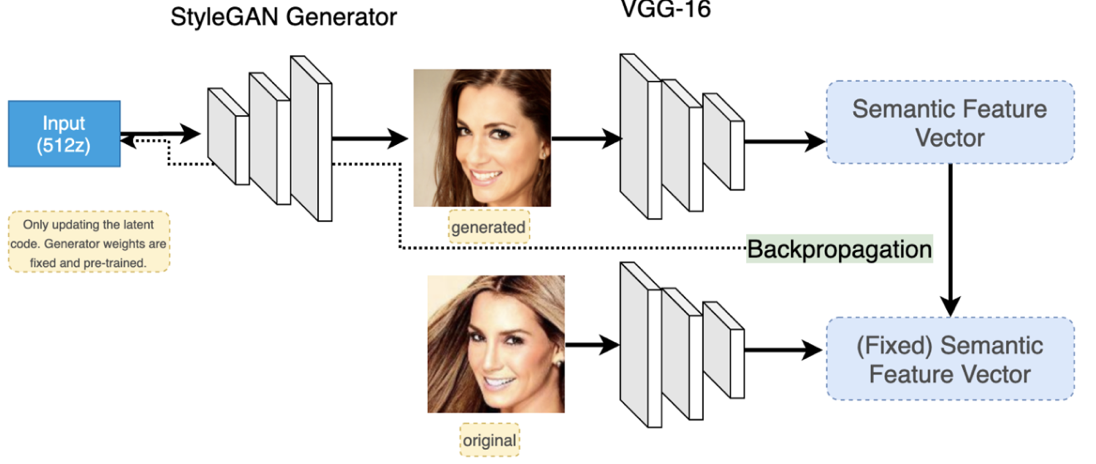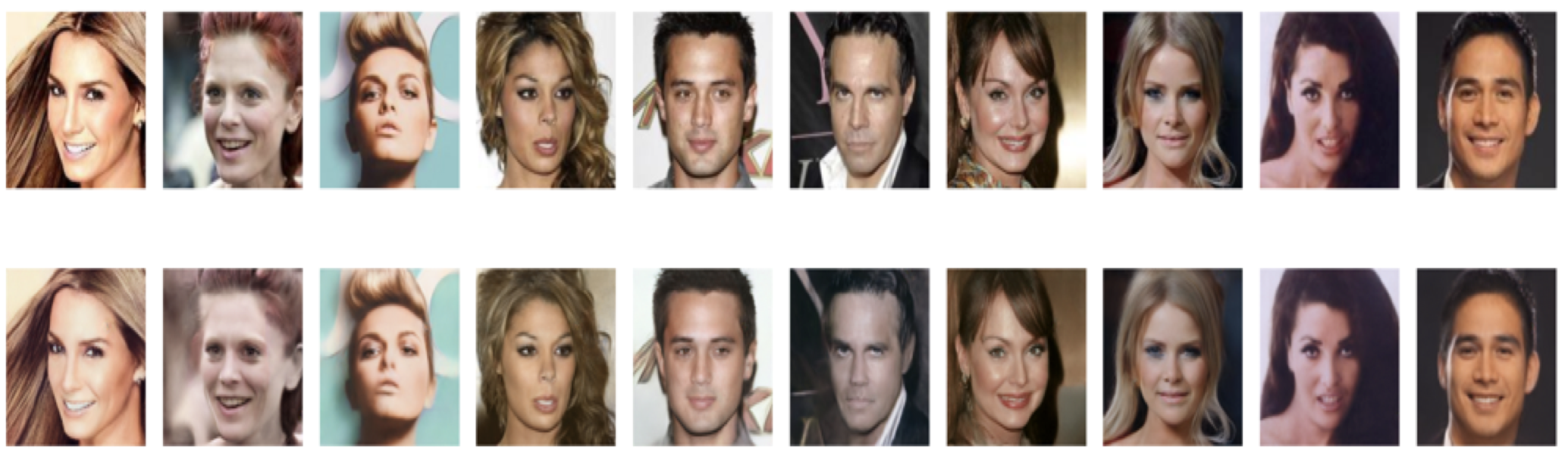# Flickr-Faces-HQ (FFHQ)

• (Karras, et al 2019) is a human faces dataset consisting of 70,000 high-quality PNG images at 1024x1024 resolution. These were used to make the pre-trained StyleGAN2 network.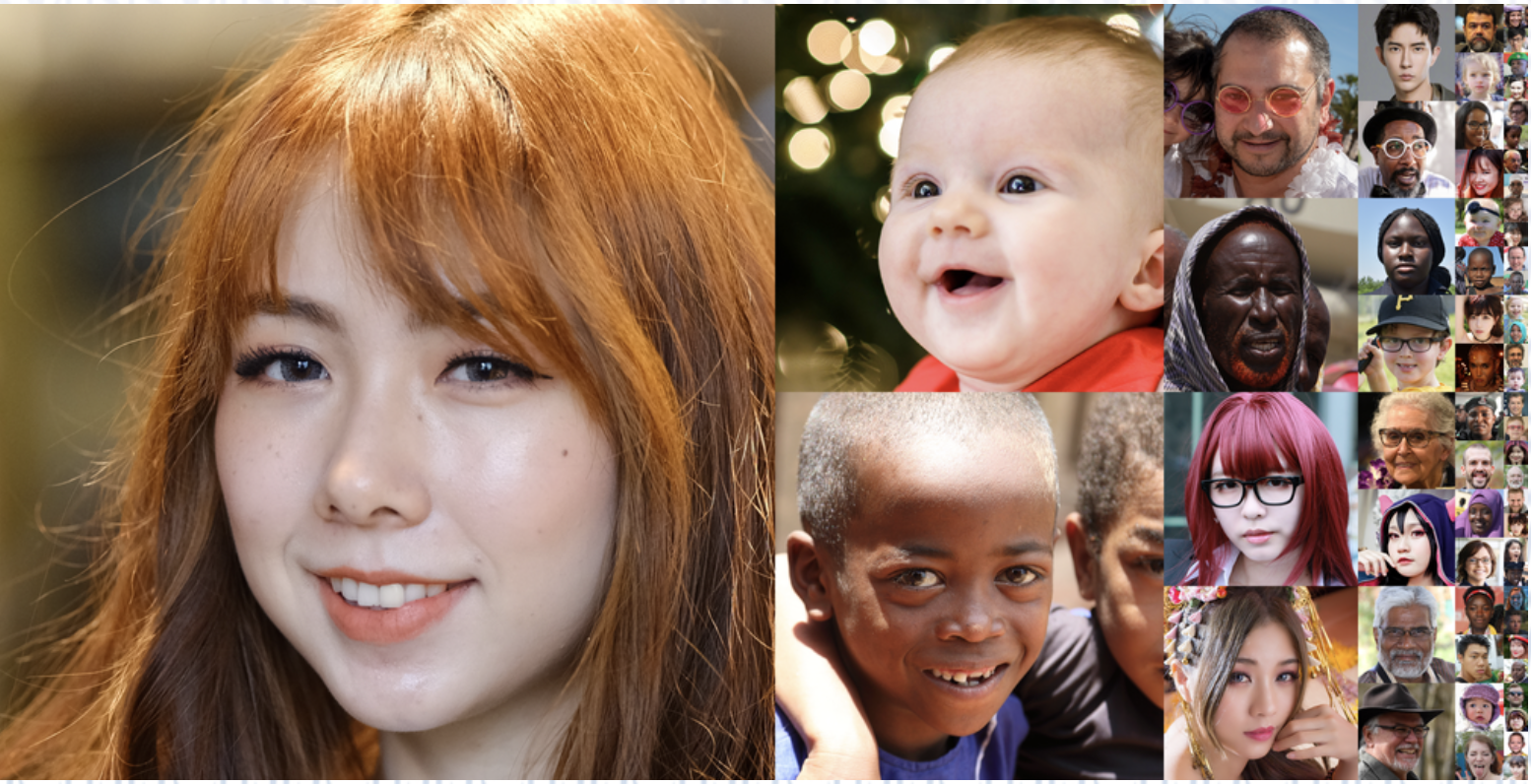# IMPA-FACE3D

• (Mena-Chalco, et al 2008) consists of 534 static images from 30 people with 6 samples of human facial expressions, 5 samples of mouth and eyes open and/or closed, and 2 samples of lateral profiles.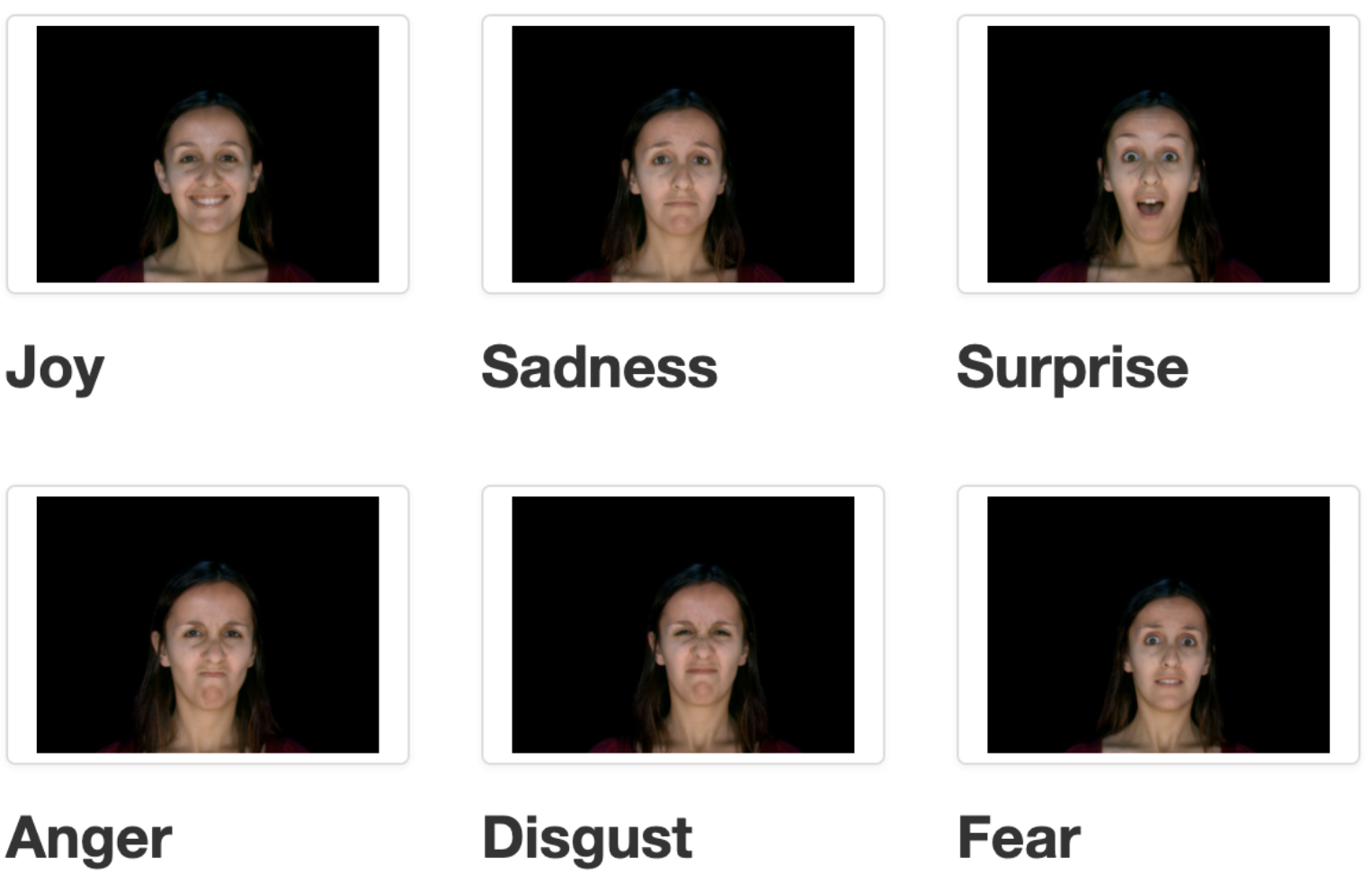# Training Emotion Directions

• We tried both a logistic regression and SVM approach to this and chose the former as it had a shorter training time.
• For each emotion, a logistic model, p(vec{x}) = 1/(1 + e^{-(vec{beta} vec{x}))), was trained on pairs (latent face codes, facial expression), to give a model that predicts the degree to which a face expresses a given emotion.
• The resulting trained vec beta was then linearly applied to a latent vector vec w for a face to control the degree to which it expressed that emotion.
• To preserve "faceness" of image, masking was used so that only 8 out of 18 of the 512 dimension vectors in vec {w} modified.
•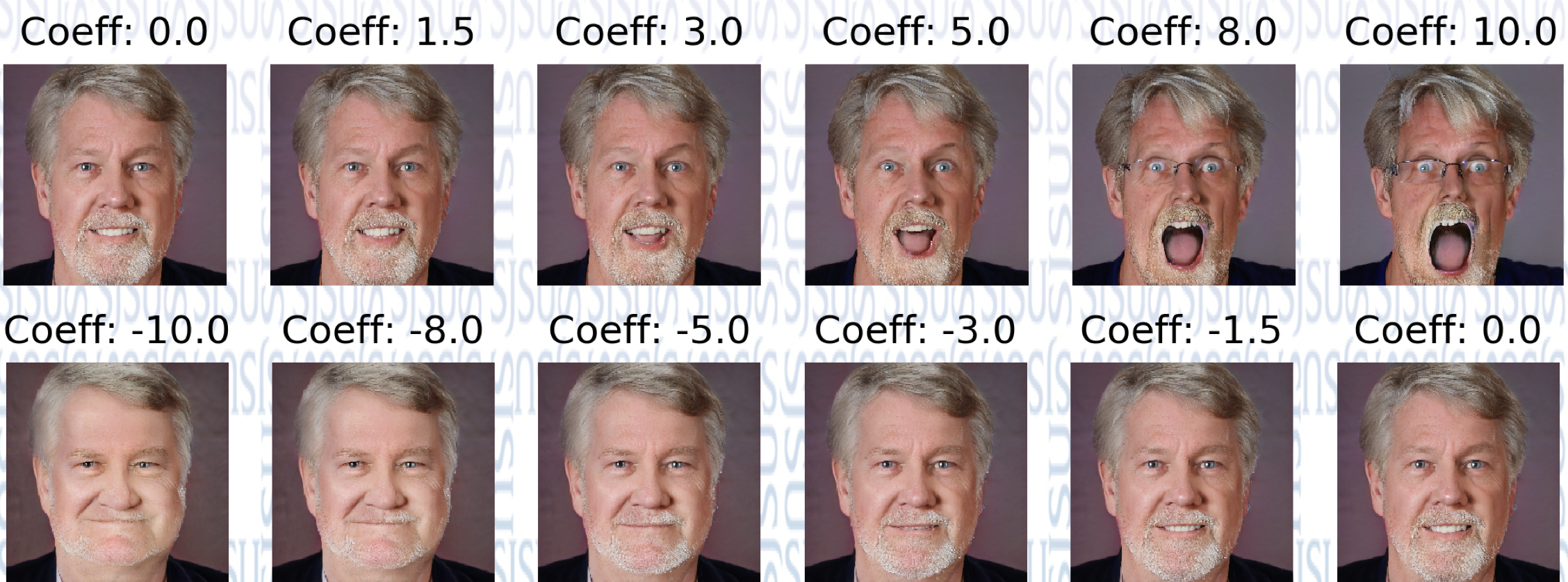# Predict Emotion Sequences

• Then used pre-trained EmoPy model to extract faces and emotions from clip.
• Trained an LSTM based model to predict next emotion based on images and priror emotions.

# Keyframes and Interpolation

• We used the following procedure to generate keyframes for our videos:
1. Randomly generate latent space vector.
2. Generate face from latent space vector.
3. Predict emotion, generate emotion sequence.
4. Repeat through sequence generating frames:
• Using the latent space vector, use vec{beta} for emotion and a fixed coefficient choice to generate a face with that emotion.
• After generating keyframe latent vectors K_i, we linearly interpolate latent vectors I = tK_i + (1-t)K_{i+1} and then generate images.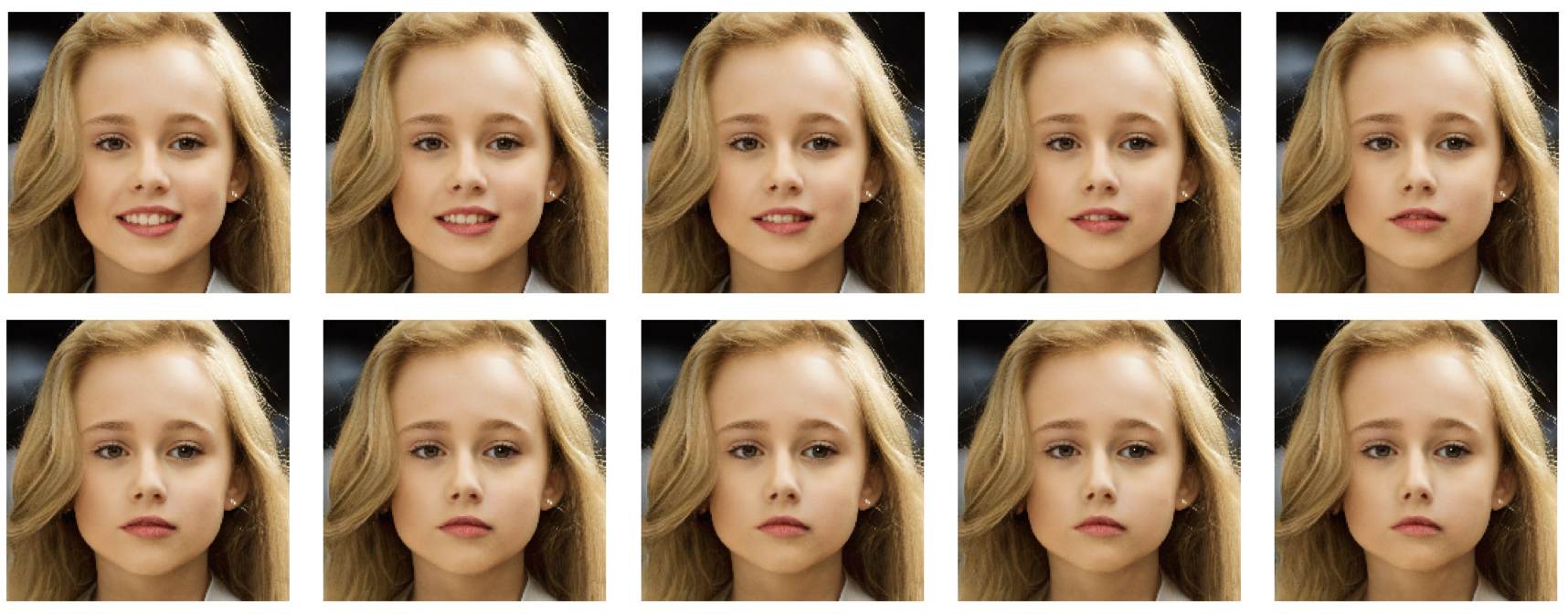# Experiments

• To evaluate generated video we followed the MocoGAN paper and looked at Average Content Distance (ACD) for facial expression videos we generated as compared to other systems.
• For facial expressions, the MocoGAN paper calculates ACD as the average L2 distance of the per-frame feature vectors from OpenFace (Schroff 2015).
• The numbers below for TGAN and MocoGAN are from the MocoGAN paper where they generated 256 videos each of 16 frames, each video representing carrying out one emotion from a list of six.
• For our experiments, we generated 256 videos from 43 randomly generated faces each of 16 frames, each video represents again carrying out one emotion.
• A smaller ACD score is better which means a generated video is more likely to be of the same person.
ModelACD
TGAN0.305
MoCoGAN0.201
Our Model0.167

# Conclusion

We conclude this talk with some observations based on our experiments with our video generation model:
• Both TGAN and MocoGAN approaches train on videos and so can work provided you have a suitable training set of videos.
• Our technique operates on a trained StyleGAN-like model of a suitable collection of images provided we have a high-level known set of action images.
• We can then train models that generate high-level action sequences and apply our technique to make a video.
• Alternatively, we can make videos from scripted sequence of high-level actions (the particular case we showed was for facial expression of emotion).
• As our technique is closer to morphing, we can make longer sequences before the frames become not humanly plausible.
• We are also able to generate high resolution video (1024 x 1024) on a single machine albeit with a high end graphics card.

# References

 M. Saito, E. Matsumoto, and S. Saito, "Temporal generative adversarial nets with singular value clipping," Proceedings of the IEEE International Conference on Computer Vision (ICCV), 2017, pp. 2830--2839.

 R. Abdal, Y. Qin, and P. Wonka, "Image2StyleGAN: How to Embed Images Into the StyleGAN Latent Space?," Proceedings of the IEEE International Conference on Computer Vision. 2019.

 T. Karras, et al., "Progressive growing of GANS for improved quality, stability, and variation," International Conference on Learning Representations (ICLR), 2018.

 S. Tulyakov, et al., "MoCoGAN: Decomposing Motion and Content for Video Generation," 2018 IEEE/CVF Conference on Computer Vision and Pattern Recognition, 2018. pp. 1526--535, doi: 10.1109/CVPR.2018.00165.

 T. Karras, S. Laine, and T. Aila, "A style-based generator architecture for generative adversarial networks," 2019 IEEE/CVF Conference on Computer Vision and Pattern Recognition (CVPR), 2019, pp. 4396--4405, doi: 10.1109/CVPR.2019.00453.

 N. Aifanti, C. Papachristou, and A. Delopoulos, "The MUG facial expression database," 11th International Workshop on Image Analysis for Multimedia Interactive Services WIAMIS 10. IEEE, 2010.

 T. Karras, et al., "Analyzing and improving the image quality of StyleGAN," 2020 IEEE/CVF Conference on Computer Vision and Pattern Recognition (CVPR), pp. 8107-8116.

 S. Ji, et al., "3D convolutional neural networks for human action recognition," IEEE Transactions on Pattern Analysis and Machine Intelligence, 35(1):221--231, 2013.